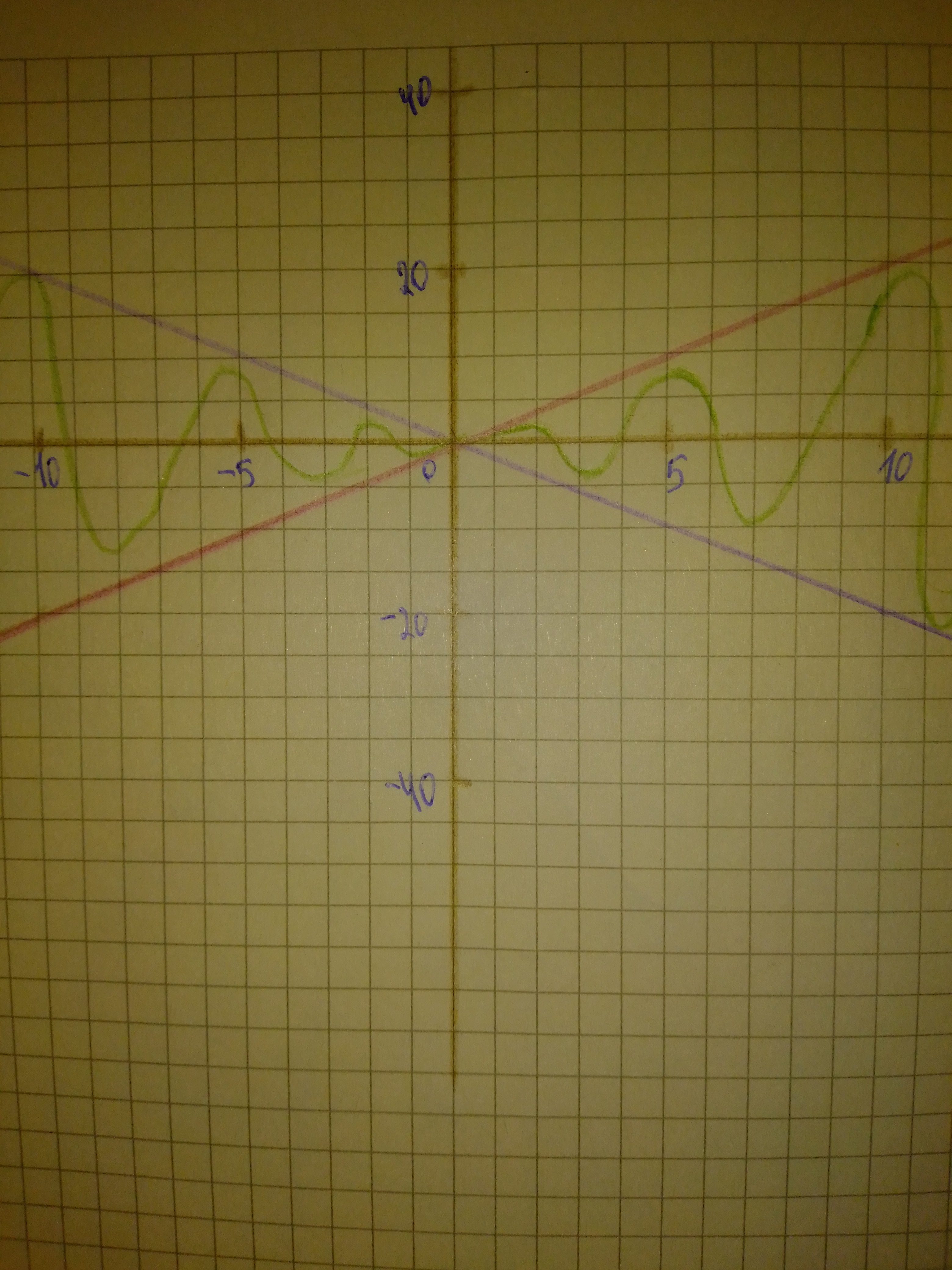# Investigate the change in the graph of a sinusoidal function of the form and=sin x or and=cos x when multiplied by a polynomial function. Use a graphing calculator to sketch the graphs of and =2x, and =-2x, and=2x cos x on the same coordinate plane, on the interval [-20, 20].Question
Polynomial graphsInvestigate the change in the graph of a sinusoidal function of the form $$\displaystyle{\quad\text{and}\quad}={\sin{{x}}}{\quad\text{or}\quad}{\quad\text{and}\quad}={\cos{{x}}}$$ when multiplied by a polynomial function. Use a graphing calculator to sketch the graphs of and $$\displaystyle={2}{x},{\quad\text{and}\quad}=-{2}{x},{\quad\text{and}\quad}={2}{x}{\cos{{x}}}$$ on the same coordinate plane, on the interval $$\displaystyle{\left[-{20},{20}\right]}.$$2021-02-23
Step 1### Relevant QuestionsUsing calculus, it can be shown that the arctangent function can be approximated by the polynomial
$$\displaystyle{\arctan{\ }}{x}\ \approx\ {x}\ -\ {\frac{{{x}^{{{3}}}}}{{{3}}}}\ +\ {\frac{{{x}^{{{5}}}}}{{{5}}}}\ -\ {\frac{{{x}^{{{7}}}}}{{{7}}}}$$
a) Use a graphing utility to graph the arctangent function and its polynomial approximation in the same viewing window. How do the graphs compare?
b) Study the pattern in the polynomial approximation of the arctangent function and predict the next term. Then repeat part (a). How does the accuracy of the approximation change when an additional term is added?In calculus, it can be shown that the arctangent function can be approximated by the polynomial
$$\displaystyle{\arctan{{x}}}\approx{x}-\frac{{x}^{{{3}}}}{{3}}+\frac{{x}^{{{5}}}}{{5}}-\frac{{x}^{{{7}}}}{{7}}$$
where x is in radians. Use a graphing utility to graph the arctangent function and its polynomial approximation in the same viewing window. How do the graphs compare?Using calculus, it can be shown that the secant function can be approximated by the polynomial $$\displaystyle{\sec{{x}}}\approx{1}+{\frac{{{x}^{{{2}}}}}{{{2}!}}}+{\frac{{{5}{x}^{{{4}}}}}{{{4}!}}}$$ where x is in radians. Use a graphing utility to graph the secant function and its polynomial approximation in the same viewing window. How do the graphs compare?a) Identify the parameters a, k, d, and c in the polynomial function $$\displaystyle{y}={\frac{{{1}}}{{{3}}}}{\left[-{2}{\left({x}+{3}\right)}\right]}^{{{4}}}-{1}$$. Describe how each parameter transforms the base function $$\displaystyle{y}={x}^{{{4}}}$$. b) State the domain and range, the vertex, and the equation of the axis of symmetry of the transformed function. c) Describe two possible orders in which the transformations can be applied to the graph of $$\displaystyle{y}={x}^{{{4}}}$$ to produce the graph of $$\displaystyle{y}={\frac{{{1}}}{{{3}}}}{\left[-{2}{\left({x}+{3}\right)}\right]}^{{{4}}}-{1}$$. d) Sketch graphs of the base function and the transformed function on the same set of axes.Using calculus, it can be shown that the tangent function can be approximated by the polynomial $$\displaystyle{\tan{\ }}{x}\ \approx\ {x}\ +\ {\frac{{{2}{x}^{{{3}}}}}{{{3}!}}}\ +\ {\frac{{{16}{x}^{{{5}}}}}{{{5}!}}}$$ where x is in radians. Use a graphing utility to graph the tangent function and its polynomial approximation in the same viewing window. How do the graphs.Find the quadratic polynomial $$\displaystyle{g{{\left({x}\right)}}}-{a}{x}^{{{2}}}\ +\ {b}{x}\ +\ {c}\ \text{which best fits the function}\ {f{{\left({x}\right)}}}={e}^{{{x}}}\ \text{at}\ {x}={0},\ \text{in the sense that}\ {g{{\left({0}\right)}}}={f{{\left({0}\right)}}},\ \text{and}\ {g}'{\left({0}\right)}={f}'{\left({0}\right)},\ \text{and}\ {g}{''}{\left({0}\right)}={f}{''}{\left({0}\right)}.$$ Using a computer or calculator, sketch graphs of f and g on the same axes. What do you notice?a) Sketch graphs of $$\displaystyle{y}={\sin{\ }}{x}$$ and $$\displaystyle{y}={\cos{\ }}{x}.$$Sketch graphs of each of the following polynomial functions. Be sure to label the x- and they-intercepts of each graph. $$\displaystyle{a}.{y}={x}{\left({2}{x}+{3}\right)}{\left({2}{x}-{5}\right)}{b}.{y}={\left({11}-{2}{x}\right)}^{{{2}}}{\left({x}-{2}\right)}$$Rational functions can have any polynomial in the numerator and denominator. Analyse the key features of each function and sketch its graph. Describe the common features of the graphs. $$\displaystyle{a}{)}{f{{\left({x}\right)}}}={\frac{{{x}}}{{{x}^{{{2}}}-{1}}}}\ {b}{)}{g{{\left({x}\right)}}}={\frac{{{x}-{2}}}{{{x}^{{{2}}}+{3}{x}+{2}}}}\ {c}{)}{h}{\left({x}\right)}={\frac{{{x}+{5}}}{{{x}^{{{2}}}-{x}-{12}}}}$$(a) find the Maclaurin polynomial $$\displaystyle{P}_{{{3}}}{\left({x}\right)}$$ for f(x), (b) complete the following $$\displaystyle{x}:-{0.75},-{0.50},-{0.25},{0},{0.25},{0.50},{0.75}{f}{\quad\text{or}\quad}{f{{\left({x}\right)}}}$$ and $$\displaystyle{P}_{{{3}}}{\left({x}\right)}$$, and (c) sketch the graphs of f(x) and $$\displaystyle{P}_{{{3}}}{\left({x}\right)}$$ on the same set of coordinate axes. $$\displaystyle{f{{\left({x}\right)}}}={\arcsin{{x}}}$$